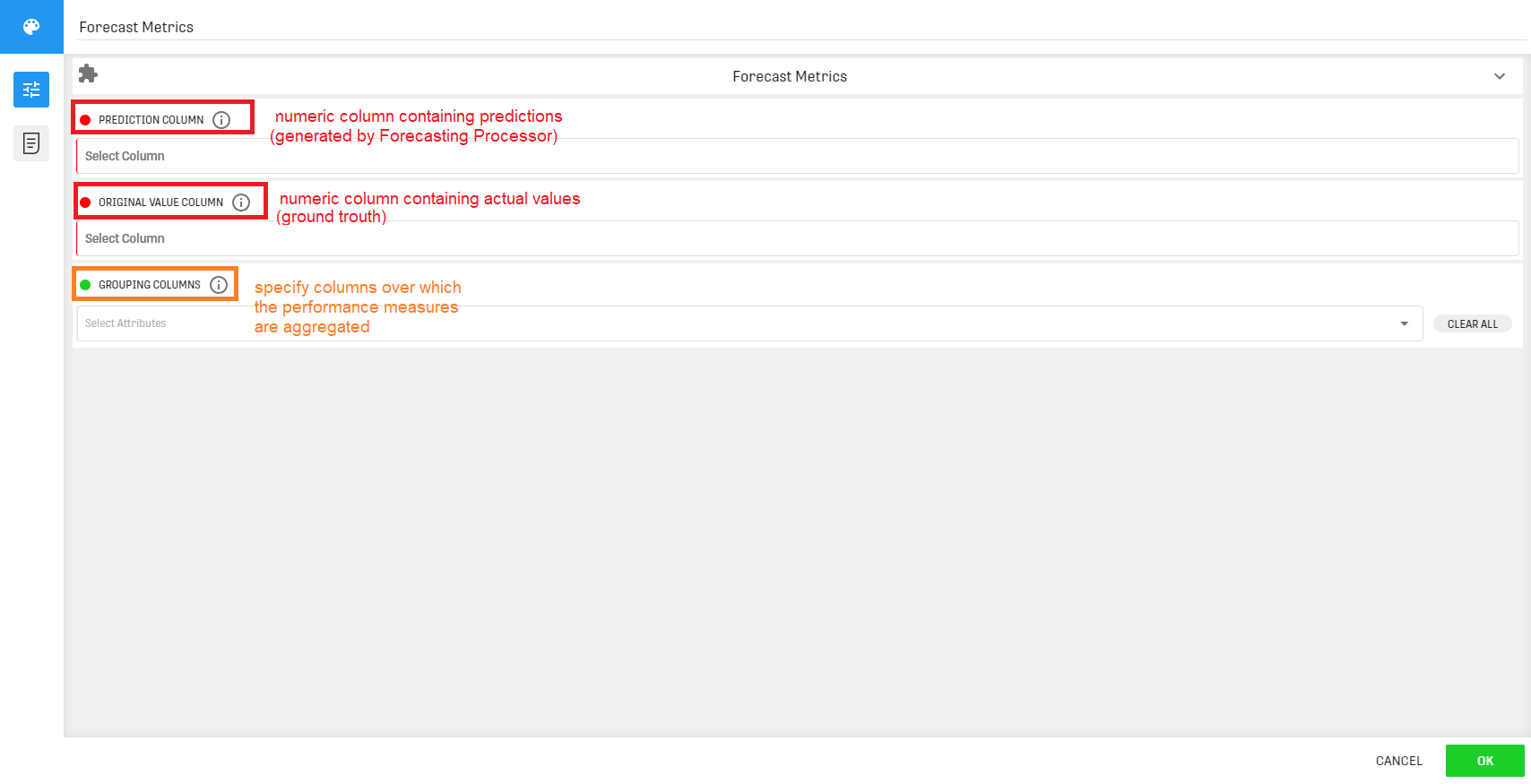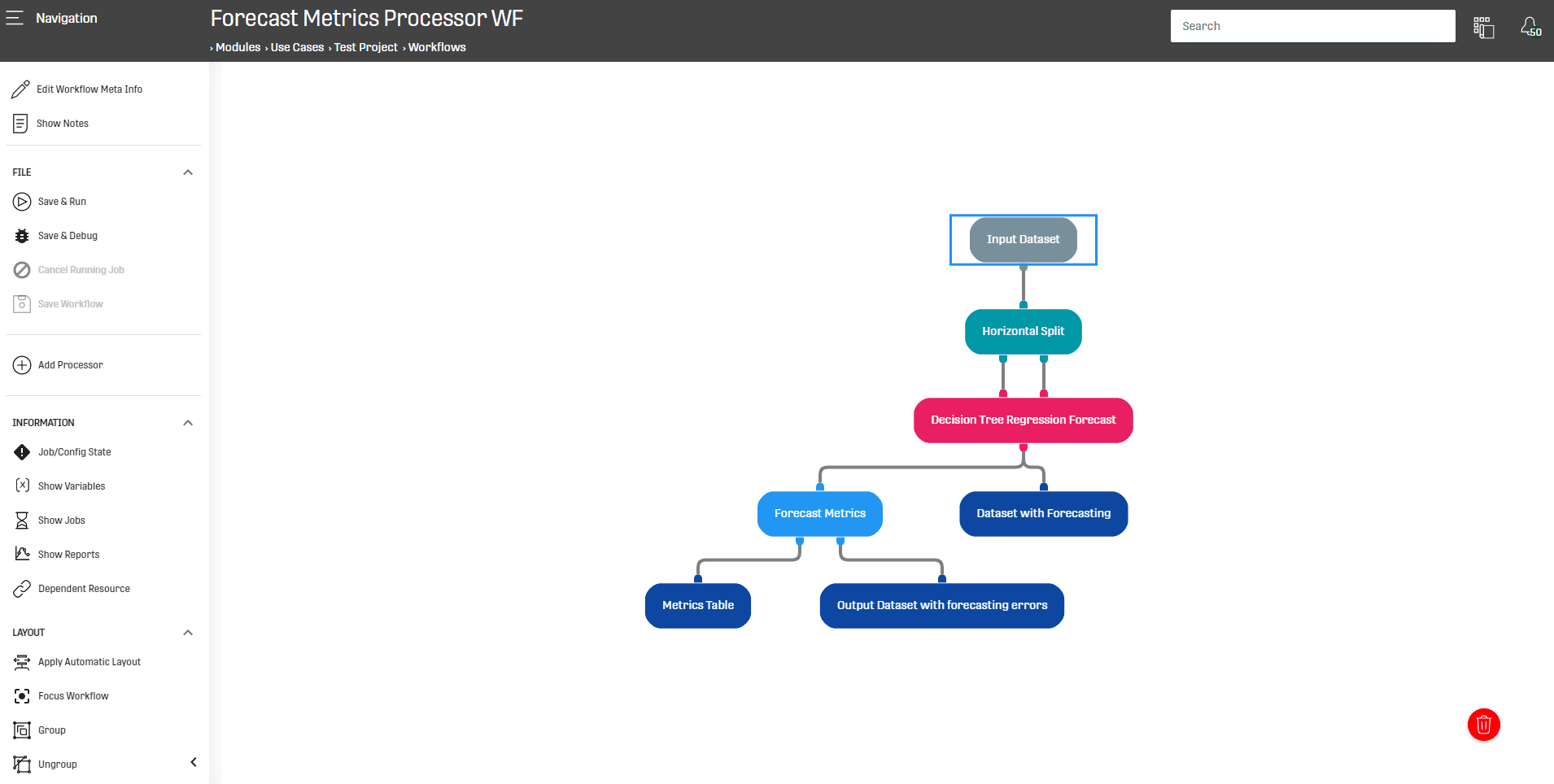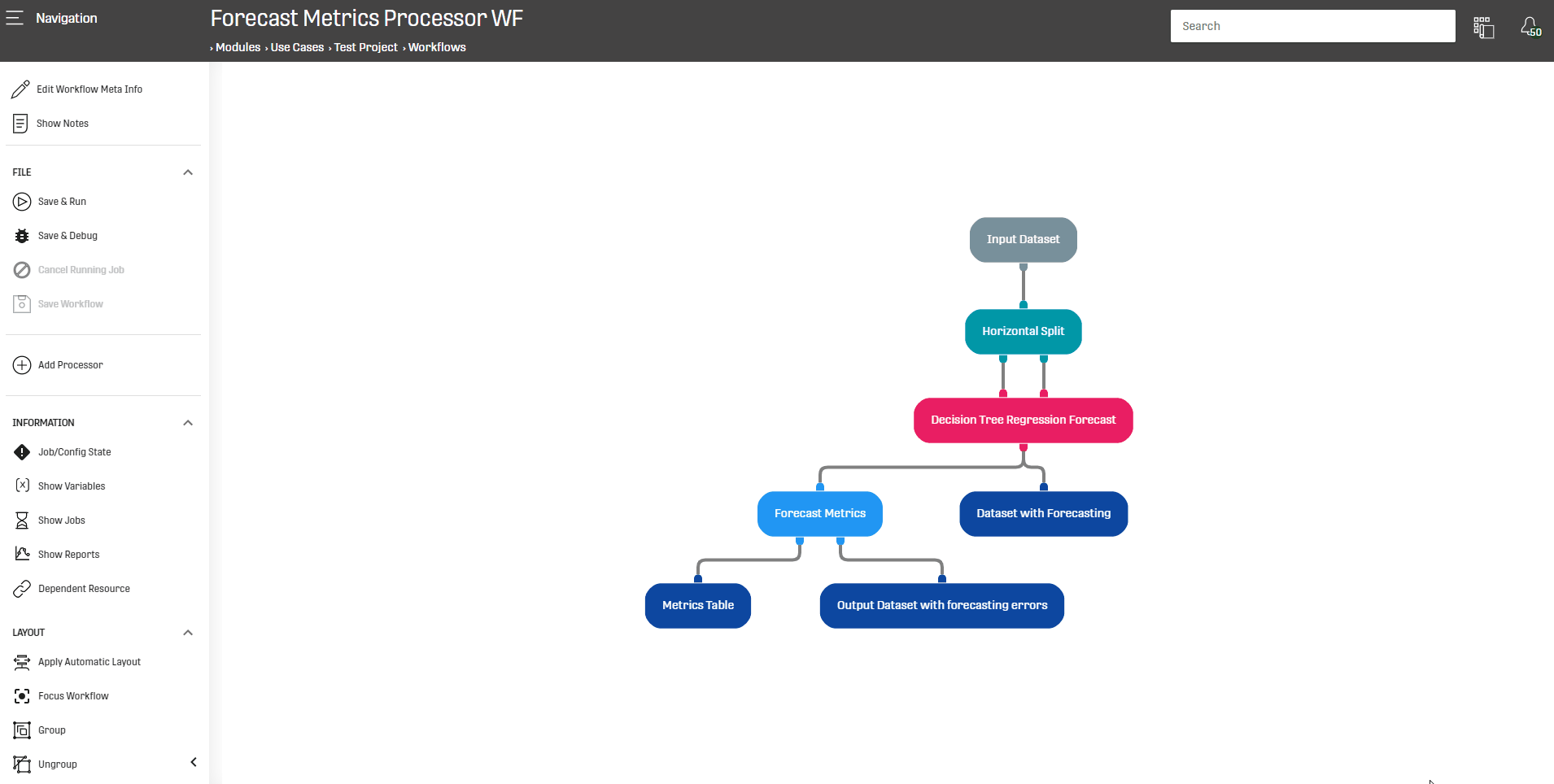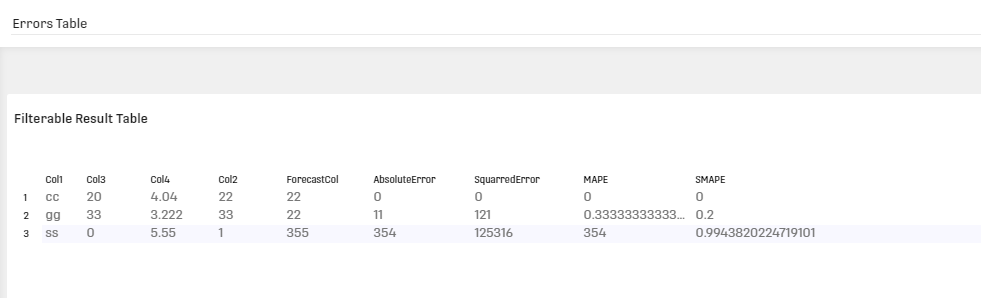# Overview

The Forecast Metrics Processor calculates different error measures for forecasting models.

# Motivation

ONE DATA includes several Processors which can be used to train a Forecasting model. These processors use sophisticated algorithms to extract patterns from input Data to use it and make predictions, and it would be helpful to have an evaluation tool for their output. Generally in Data Science evaluating the model performance is very important to determine its efficiency and discuss overfitting.

# Configuration

This processor should be generally linked to a Forecast Processor such as Decision Tree Regression Forecast Processor or a similar processors which generate a Forecasting (prediction) column. Precisely, this processor needs the original and forecast columns from the input Data.

Technically this processor can operate with any Dataset having two numeric columns, but that is not the purpose behind using this processor.

The configuration interface of the processor looks like the following:The first field refers to the column containing the original Data. The second field refers to the prediction/forecast column that is generally produced by a Forecast processor.

The Forecast Metrics Processor uses these two columns to calculate different error measures.

# Output

This processor has two output nodes:

# Example

In the following example the Forecast Metrics Processor will be used to measure the performance of a Decision Tree Regression Forecast trained on a simple Dataset:The Horizontal Split Processor was used to split the Dataset into training and test sets, then the Decision Tree Regression Forecast is trained on the second Column as dependent column and third and forth columns as independent columns (the generated forecasting are saved under the Column "ForecastCol")

The Forecast Metrics Processor will then collect the output to calculate performance metrics for the predicted column with respect to the original one.

After running the Workflow, the outputs from the Decision Tree Regression Forecast and Forecast Metrics processors can be visualised via the Result Tables :The right node of the processor will generate the following table (note that "Col2" was translated near the Forecast Column to make the comparison easier).For the first entry: the value in Col2 (Column containing original data) is the same as in the Forecasting column

=> That is why the error is null

For the second entry: Col2 has a value of 22 and the Forecasting column has a value of 33

=> The absolute error (absolute value from the difference) is then 11

# Related Articles

Decision Tree Regression Forecast

Decision Tree Classification Forecast

Random Forest Classification Forecast Processor

Random Forest Regression Forecast Processor